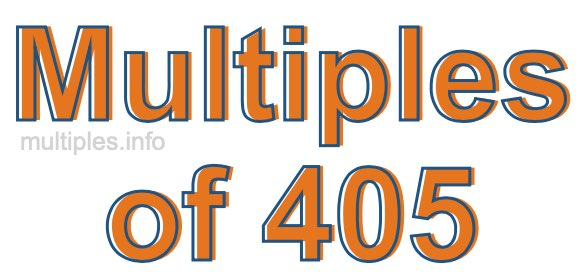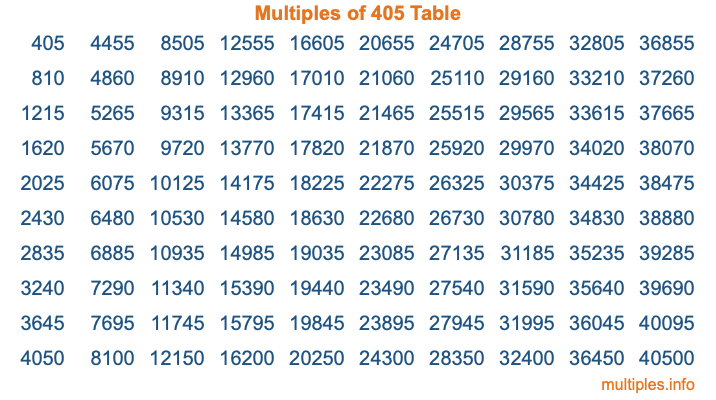Multiples of 405Welcome to the Multiples of 405 page. Here we will first teach you everything you will ever need to know about the multiples of 405, and then give you a study guide summary of everything we taught you to make sure you remember it all. Use this page to look up facts and learn information about the multiples of 405. This page will make you a multiples of four hundred five expert!

Definition of Multiples of 405
Multiples of 405 are all the numbers that when divided by 405 equal an integer. Each of the multiples of 405 are called a multiple. A multiple of 405 is created by multiplying 405 by an integer.

Therefore, to create a list of multiples of 405, you start with 1 multiplied by 405, then 2 multiplied by 405, then 3 multiplied by 405, and so on for as long as you want. Thus, the list of the first five multiples of 405 is 405, 810, 1215, 1620, and 2025. To see a larger list of multiples of 405, see the printable image of Multiples of 405 further down on this page. We also have a category where you can choose any nth multiple of 405.

Multiples of 405 Checker
The Multiples of 405 Checker below checks to see if any number of your choice is a multiple of 405. In other words, it checks to see if there is any number (integer) that when multiplied by 405 will equal your number. To do that, we divide your number by 405. If the the quotient is an integer, then your number is a multiple of 405.

Is  a multiple of 405?

Least Common Multiple of 405 and ...
A Least Common Multiple (LCM) is the lowest multiple that two or more numbers have in common. This is also called the smallest common multiple or lowest common multiple and is useful to know when you are adding our subtracting fractions. Enter one or more numbers below (405 is already entered) to find the LCM.

Check out our LCM Calculator if you need more details about the Least Common Multiple or if you need the LCM for different numbers for adding and subtraction fractions.

nth Multiple of 405
As we stated above, 405 is the first multiple of 405, 810 is the second multiple of 405, 1215 is the third multiple of 405, and so on. Enter a number below to find the nth multiple of 405.

th multiple of 405

Multiples of 405 vs Factors of 405
405 is a multiple of 405 and a factor of 405, but that is where the similarities end. All postive multiples of 405 are 405 or greater than 405. All positive factors of 405 are 405 or less than 405.

Below is the beginning list of multiples of 405 and the factors of 405 so you can compare:

Multiples of 405: 405, 810, 1215, 1620, 2025, etc.

Factors of 405: 1, 3, 5, 9, 15, 27, 45, 81, 135, 405

As you can see, the multiples of 405 are all the numbers that you can divide by 405 to get a whole number. The factors of 405, on the other hand, are all the whole numbers that you can multiply by another whole number to get 405.

It's also interesting to note that if a number (x) is a factor of 405, then 405 will also be a multiple of that number (x).

Multiples of 405 vs Divisors of 405
The divisors of 405 are all the integers that 405 can be divided by evenly. Below is a list of the divisors of 405.

Divisors of 405: 1, 3, 5, 9, 15, 27, 45, 81, 135, 405

The interesting thing to note here is that if you take any multiple of 405 and divide it by a divisor of 405, you will see that the quotient is an integer.

Multiples of 405 Table
Below is an image of the first 100 multiples of 405 in a table. The table is in chronological order, column by column. The first column has the first ten multiples of 405, the second column has the next ten multiples of 405, and so on.The Multiples of 405 Table is also referred to as the 405 Times Table or Times Table of 405. You are welcome to print out our table for your studies.

Negative Multiples of 405
Although not often discussed or needed in math, it is worth mentioning that you can make a list of negative multiples of 405 by multiplying 405 by -1, then by -2, then by -3, and so on, to get the following list of negative multiples of 405:

-405, -810, -1215, -1620, -2025, etc.

Multiples of 405 Summary
Below is a summary of important Multiples of 405 facts that we have discussed on this page. To retain the knowledge on this page, we recommend that you read through the summary and explain to yourself or a study partner why they hold true.

There are an infinite number of multiples of 405.

A multiple of 405 divided by 405 will equal a whole number.

405 divided by a factor of 405 equals a divisor of 405.

The nth multiple of 405 is n times 405.

The largest factor of 405 is equal to the first positive multiple of 405.

405 is a multiple of every factor of 405.

405 is a multiple of 405.

A multiple of 405 divided by a divisor of 405 equals an integer.

405 divided by a divisor of 405 equals a factor of 405.

Any integer times 405 will equal a multiple of 405.

Multiples of a Number
Here you can get the multiples of another number, all with the same attention to detail as we did for multiples of 405 on this page.

Multiples of
Multiples of 406
Did you find our page about multiples of four hundred five educational? Do you want more knowledge? Check out the multiples of the next number on our list!